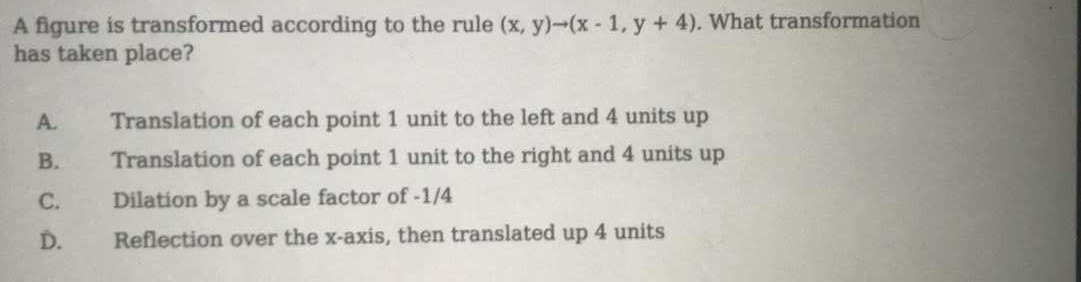### ¿Todavía tienes preguntas de matemáticas?

Pregunte a nuestros tutores expertos
Algebra
PreguntaA figure is transformed according to the rule $$( x , y ) \rightarrow ( x - 1 , y + 4 )$$ . What transformation has taken place? A. Translation of each point $$1$$ unit to the left and $$4$$ units up B. Translation of each point $$1$$ unit to the right and $$4$$ units up C. Dilation by a scale factor of $$- 1 / 4$$

D. Reflection over the x-axis, then translated up $$4$$ units